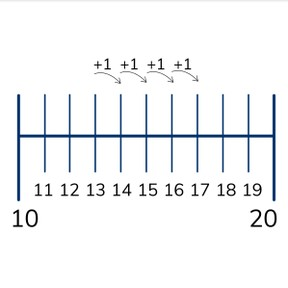Efficiently counting to a number on the number line - within 20

# Efficiently counting to a number on the number line - within 208,000 schools use Gynzy92,000 teachers use Gynzy1,600,000 students use Gynzy

## General

Students learn to count up to a number max 20 on a number line. They learn to count in jumps to reach the desired number.

-K.CC.A.1
-K.CC.A.2
-K.CC.A.3

## Relevance

It is useful to learn how to count up to a number in jumps on the number line because you then learn to count how many more you need to add to a number to reach a second number.

## Introduction

The interactive whiteboard shows a number line to 20 with the letters A, B, and C. Students write down the numbers shown by the letters.

## Development

The interactive whiteboard shows a number line that shows the number 2, and students are asked to jump to 7. Explain that they are going to see how many more you need to reach 7. You can do this in five jumps of 1, but also in one jump of 5. Show the jumps by drawing them and writing the total at the top. Repeat this with jumping from 8 to 14 and from 4 to 13. Emphasize that when the number is bigger than 10 that it is useful to jump to 10 and then continue jumping.

To check that students understand counting up to a number on the number line you can ask the following questions:
- What are clever ways to count up to a number on a number line?
- If your number is bigger than ten, what is a smart thing to do?
- How do you jump from 7 to 14?

## Guided practice

Students first practice counting on the number line from one number below ten to another nubmer below ten. Then from a number above ten to one above ten and finally from a number below ten to one above ten. They can decide which jumps to use themselves. Encourage them to take bigger, and clever jumps to reach their numbers, like making ten and then counting on.

## Closing

The interactive whiteboard shows a number line with the number 7, and students are asked to jump to 19. Students can draw the number line and draw which jumps they think they should take. Hold them up to check them as a class.

## Teaching tips

If students have difficulty with counting up to a number with jumps on the number line, have them practice placing numbers in order on the number line to 20.

### The online teaching platform for interactive whiteboards and displays in schools

• Save time building lessons

• Manage the classroom more efficiently

• Increase student engagement